March 9, 2018

## Stick-people can explain things well

Despite being easy to draw, stick people are useful

We like seeing people in stories

They make the message more personal

## Example: explaining “Impostor Syndrome”

### XKCD Comic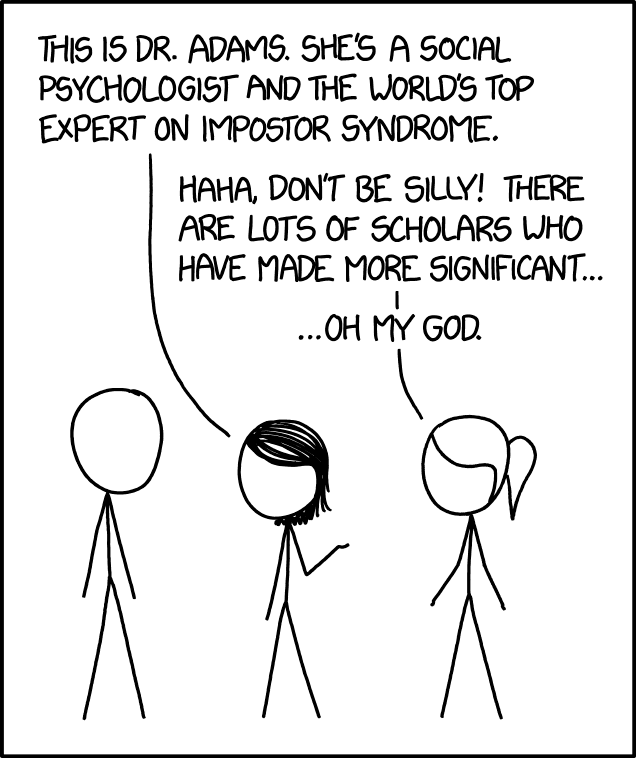## Example: explaining areas of Science

### XKCD Comic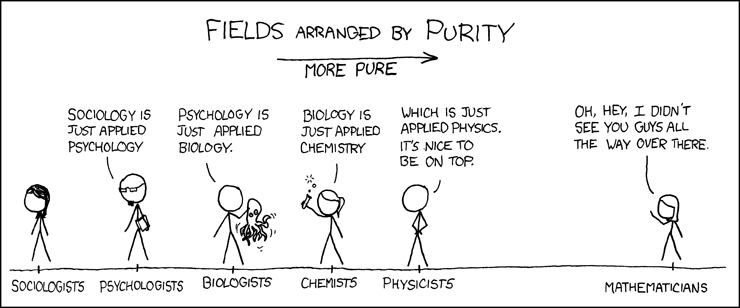## There is an xkcd library for R

### Creative comics with real data

library(xkcd)
ratioxy <- diff(range(gb$Release))/diff(range(gb$WGS.Bases))
axes <- xkcdaxis(range(gb$Release), range(gb$WGS.Bases))
axes[]$text$family <- "Humor Sans"

man1 <- xkcdman(aes(x = 140, y = 5.0e+11, scale = 8e+10, ratioxy,
angleofspine = -1.704265, anglerighthumerus = -0.5807903,
anglelefthumerus = 3.941945, anglerightradius = 0.0441480,
angleleftradius = 3.222387, anglerightleg = 5.274786,
angleleftleg = 4.349295, angleofneck = -1.820286), data=NULL)

man2 <- xkcdman(aes(x = 196, y = 2.8e+11, scale = 8e+10, ratioxy,
angleofspine = -1.389649, anglerighthumerus = -0.2829418,
anglelefthumerus = 3.379656, anglerightradius = 0.6164104,
angleleftradius = 3.073443, anglerightleg = 5.116607,
angleleftleg = 4.316328, angleofneck = -1.319579), data=NULL)

ggplot(gb, aes(Release,WGS.Bases,label="0")) +
geom_text(family="Humor Sans", alpha=0.8) + axes + man1 + man2 +
theme(plot.background = element_blank()) +
annotate("text", x=160, y=75e10, family="Humor Sans",
label="Genbank data\nkeeps growing!") +
xkcdline(aes(xbegin=145, ybegin=5e11, xend=165, yend=65e10),
data=NULL, xjitteramount = 10)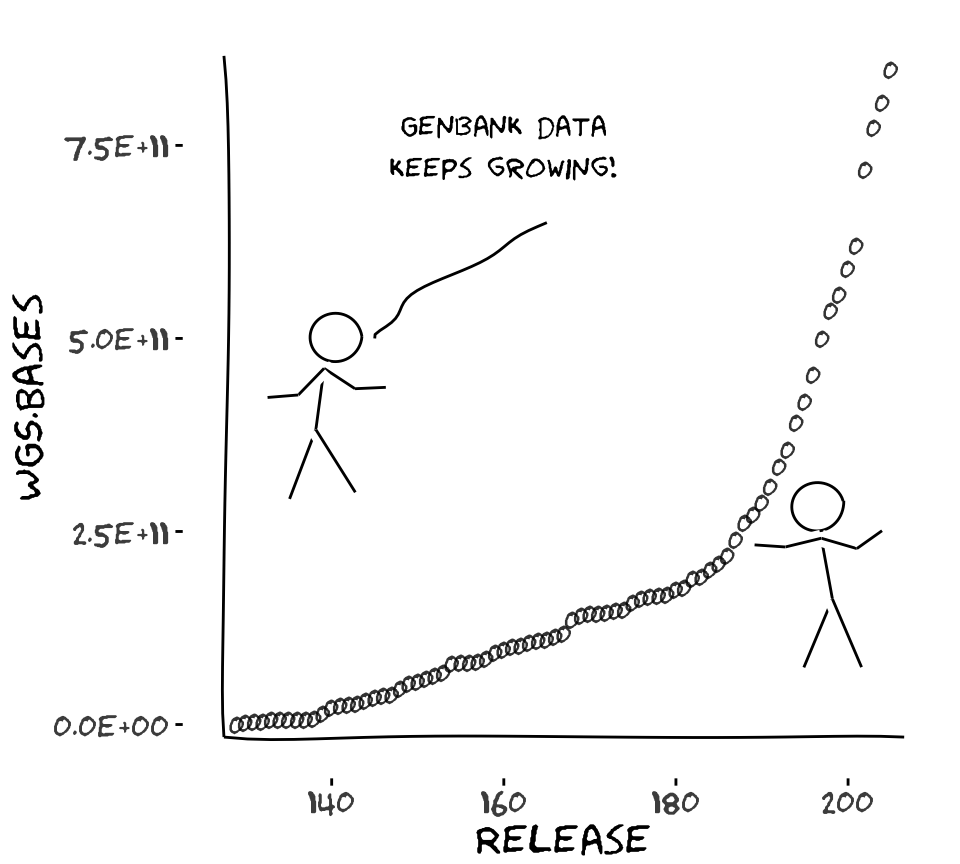## Faces can illustrate complex data

### Invented by Herman Chernoff in 1973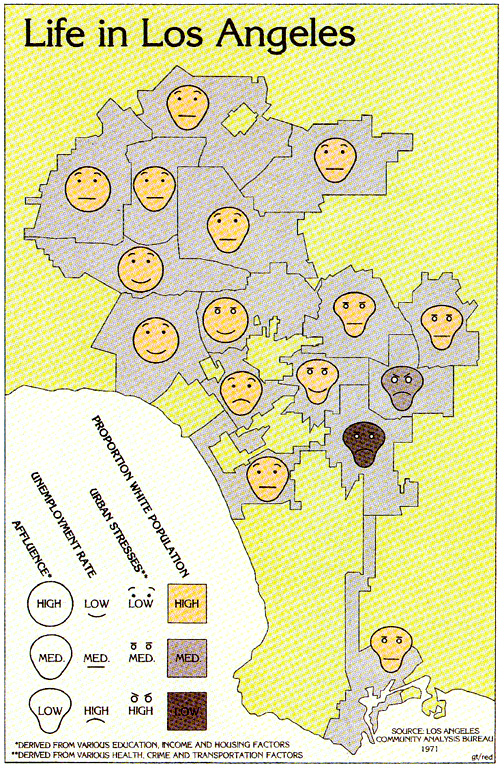• Show data in the shape of a human face
• Eyes, ears, mouth and nose represent values by their shape, size, placement and orientation
• Humans easily recognize faces and easily notice small changes
• The mapping of features should be careful
• eye size and eyebrow can have significant weight

## Comics can be used to communicate science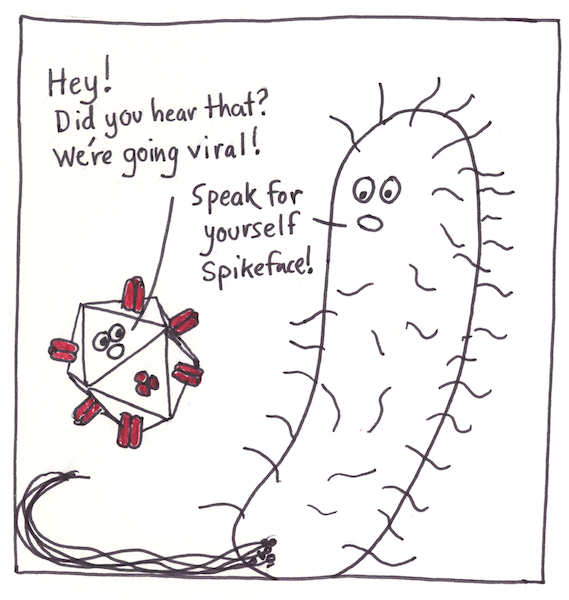This paper has just been published:

Ten simple rules for drawing scientific comics.
McDermott JE, Partridge M, Bromberg Y
PLoS Computational Biology 14(1) (2018): e1005845.

## It is easy if you know the Accession Number

Accession numbers are the best way to identify a biological sequence

• Candidatus Carsonella ruddii PV DNA has accession AP009180
• Escherichia coli str. K-12 substr. MG1655 has accession NC_000913

Different sequences can have the same name, but never the same accession

## If you do not know the accession use Taxonomy

NCBI search box looks for patterns everywhere, not only on the title

and many sequences can have the same name

To be sure of using the correct sequence, go to NCBI Taxonomy

https://www.ncbi.nlm.nih.gov/taxonomy

Try with “Escherichia coli”. There are many

## Once you find the correct species…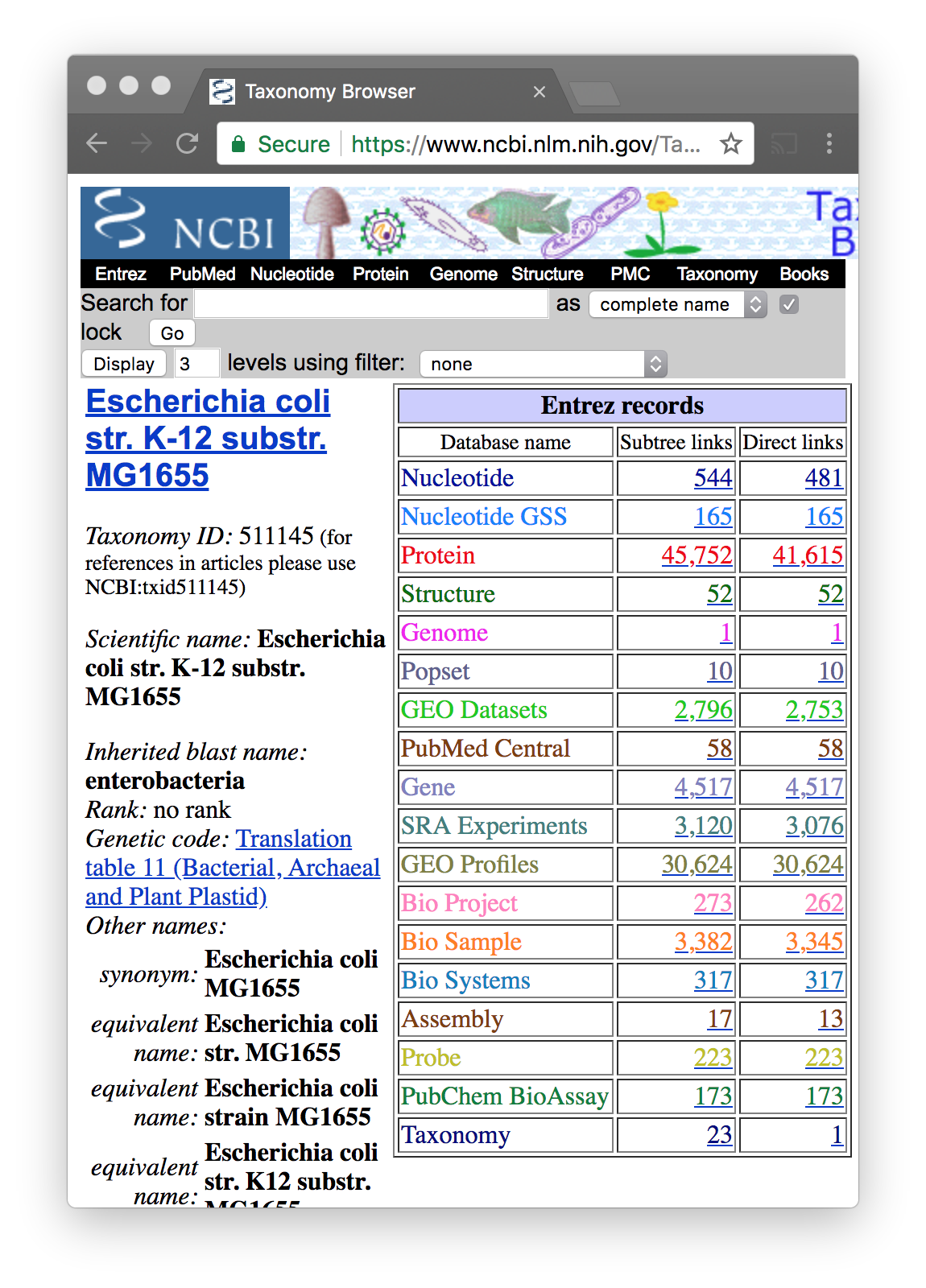You will see that each organism has a taxonomic ID

In the search box add the phrase “complete genome”

## When you find the correct one..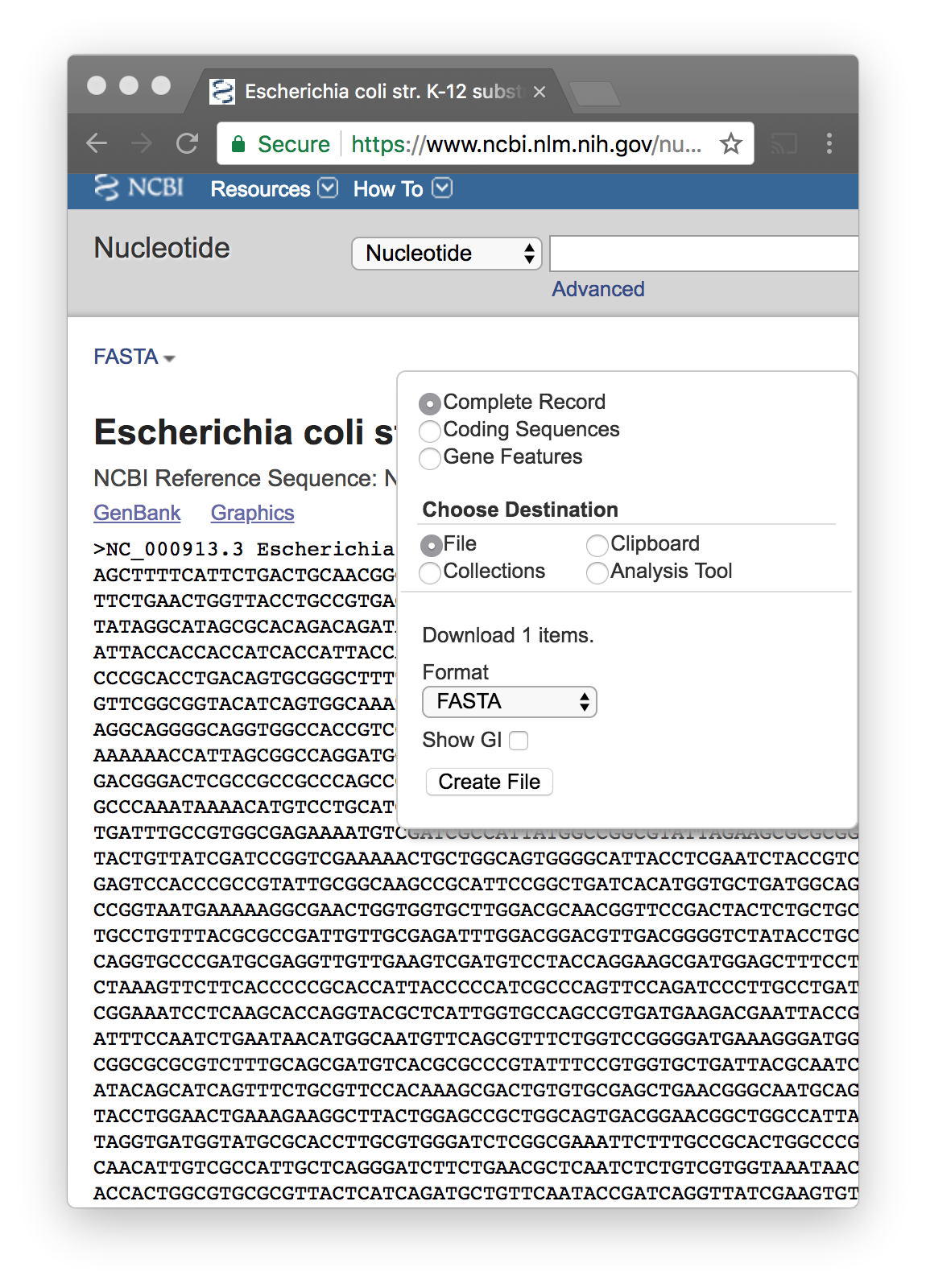Store it on your computer, and change the name

If you use the Lab’s computers, save it on X:

To handle sequence data in R, we use the seqinr library

You have to install it once.

install.packages("seqinr")

Then you have to load it on every session

library(seqinr)

read.fasta(file, seqtype = "DNA", ...)

### Inputs

file
The name of the file which the sequences in FASTA format are to be read from
seqtype
the nature of the sequence: DNA or AA, defaulting to DNA

### Output

A list of vectors of chars. Each element is a sequence object.

## Example

library(seqinr)
dna <- sequences[]

## What have we learned?

• Some organisms have been sequenced
• We can read the sequences from FASTA files
• read.fasta returns a list of vectors of characters
• We can create functions to do things with the sequence
• Functions are black boxes with inputs and outputs
• For example we do statistics of the sequence

## Why we do statistics on the sequence?

Statistics is a way to tell a story that makes sense of the data

In genomics, we look for biological sense

That story can be global: about the complete genome

Or can be local: about some region of the genome

## GC-content

The percentage of nitrogenous bases on a DNA molecule that are either guanine or cytosine.

• GC content is found to be variable with different organisms.
• The committee on bacterial systematics has recommended use of GC ratios in higher level hierarchical classification

## GC-content can be measured by several means

Measuring the melting temperature of the DNA double helix using spectrophotometry

• The absorbance of DNA at a wavelength of 260nm increases when DNA separates into two single strands at melting temperature

## Determination of GC content

If the DNA has been sequenced then the GC-content can be accurately calculated by simple arithmetic.

GC-content percentage is calculated as $\frac{G+C}{A+T+G+C}$

## Exercise 1

Determine the GC content of E.coli

Is this GC content uniform through all genome?

## Chargaff’s rules

### Discovered by Austrian chemist Erwin Chargaff in 1952

DNA from any cell of all organisms has a 1:1 ratio of pyrimidine and purine bases

The amount of guanine is equal to cytosine and the amount of adenine is equal to thymine.

Elson D, Chargaff E (1952). On the deoxyribonucleic acid content of sea urchin gametes. Experientia 8 (4): 143–145

Chargaff E, Lipshitz R, Green C (1952). Composition of the deoxypentose nucleic acids of four genera of sea-urchin. J Biol Chem 195 (1): 155–160

## First Chargaff’s parity rule

A double-stranded DNA molecule globally has percentage base pair equality:

$$\%A = \%T$$ and $$\%G = \%C$$

The rigorous validation of the rule constitutes the basis of Watson-Crick pairs

## Second Chargaff’s parity rule

Both $$\%A \approx \%T$$ and $$\%G \approx \%C$$ are valid for each of the two DNA strands

This describes only a global feature of the base composition in a single DNA strand

## Exercise 2

Why the first rule is always valid?

How do you determine if $$\%G \approx \%C$$?

Is this valid through all the genome?

## GC Skew

What is the difference $$G-C$$ respect to the total $$G+C$$?

Calculate the ratio $\frac{G-C}{G+C}$ using sliding windows and plot it

What do you see?

## Chargaff second rule

In each DNA strand the frequency of occurrence of G is equal to C because the mutation rate is the same

Hence, the second parity rule only exists when there is no mutation or substitution

There is a richness of G over C and T over A in the leading strand

• and vice versa for the lagging strand

GC skew changes sign at the boundaries of the two replichores

This corresponds to DNA replication origin or terminus

## What is the story told by GC skew?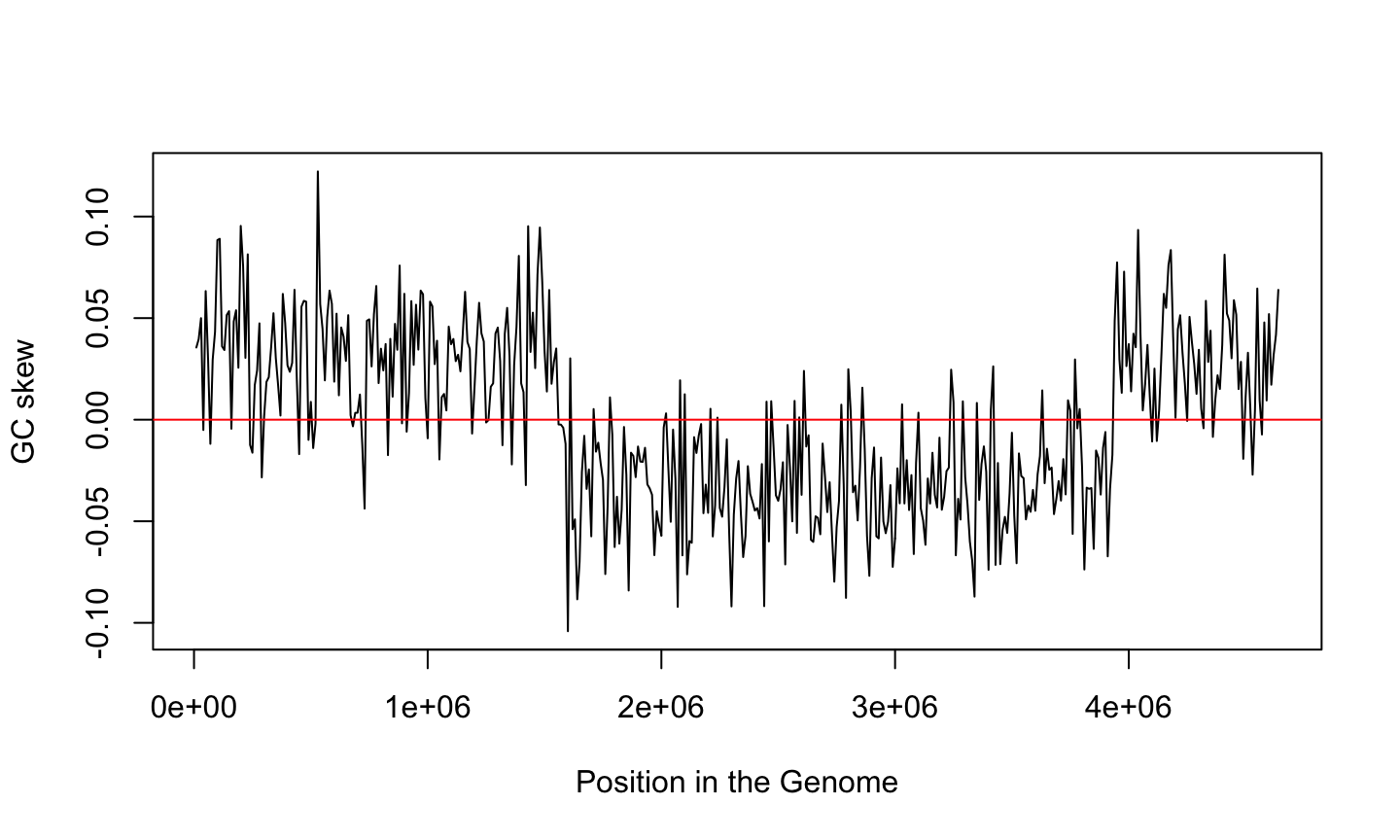## Remember Chargaff second rule

For each DNA strand we have $$\%A \approx \%T$$ and $$\%G \approx \%C$$

This is because the substitution rate is presumably equal

Hence, the second parity rule only exists when there is no mutation or substitution

But the ratio of G over C is not uniform over the genome

Why?

## GC skew and genome replication

GC skew changes sign at the boundaries of the two replichores

This corresponds to DNA replication origin or terminus

The replication origin is usually called ori.

## How to calculate values on regions

All our calculations are done using functions

What should be

• the output?
• the input(s)?
• the name?

## Defining a GC skew function

Remember that an R function is defined like this

name <- function(input1, input2, ...) {
Calculate
return(output)
}

## The inputs

The GC skew result should depend on:

• the genomic sequence
• the start of the window
• the length of the window

These are the parameters of the function. Do they have

• a name?
• a default value?

## The output

How do we transform the input parameters into the output value?

We can use any R function available, such as

seq(from, to, by, length_out)

Task: write a gc_skew function

## Applying the function to many values

We want to evaluate gc_skew on different positions of the genome

positions <- seq(from=1, to=length(dna), by= window_size)

How do we apply gc_skew to each element of positions?